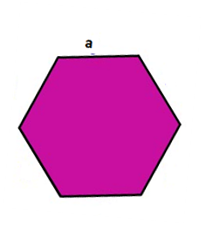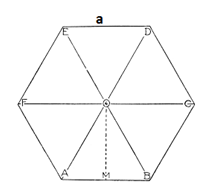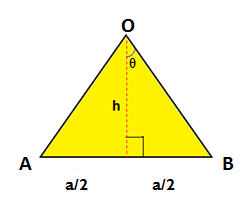# Hexagon Formula

A polygon is a two-dimensional (2-D) closed figure made up of straight line segments. In geometry, hexagon is a polygon with 6 sides. If the lengths of all the sides and the measurement of all the angles are equal, such hexagon is called a regular hexagon. In other words, sides of a regular hexagon are congruent.

There is a predefined set of formulas for the calculation of perimeter and area of a regular hexagon which is collectively called as hexagon formula. The hexagon formula for a hexagon with the side length of a, is given as:Perimeter of an Hexagon = 6a

Area of an Hexagon =

$$\begin{array}{l}\frac{3\sqrt{3}}{ 2} \times a^{2}\end{array}$$

Hexagon formula helps us to compute the area and perimeter of hexagonal objects. Honeycomb, quartz crystal, bolt head, Lug/wheel nut, Allen wrench, floor tiles etc are few things which you would find a hexagon.

Properties of a Regular Hexagon:

• It has six sides and six angles.
• Lengths of all the sides and the measurement of all the angles are equal.
• The total number of diagonals in a regular hexagon is 9.
• The sum of all interior angles is equal to 720 degrees, where each interior angle measures 120 degrees.
• The sum of all exterior angles is equal to 360 degrees, where each exterior angle measures 60 degrees.

Derivation:

Consider a regular hexagon with each side a units.

Formula for area of a hexagon: Area of a hexagon is defined as the region occupied inside the boundary of a hexagon.

In order to calculate the area of a hexagon, we divide it into small six isosceles triangles. Calculate the area of one of the triangles and then we can multiply by 6 to find the total area of the polygon.Take one of the triangles and draw a line from the apex to the midpoint of the base to form a right angle. The base of the triangle is a, the side length of the polygon. Let the length of this line be h.The sum of all exterior angles is equal to 360 degrees. Here, ∠AOB = 360/6 = 60°

∴ θ = 30°

We know that the tan of an angle is opposite side by adjacent side,

Therefore,

$$\begin{array}{l} tan\theta = \frac{\left ( a/2 \right )}{h}\end{array}$$

$$\begin{array}{l}tan30 = \frac{\left ( a/2 \right )}{h}\end{array}$$

$$\begin{array}{l}\frac{\sqrt{3}}{3}= \frac{\left ( a/2 \right )}{h}\end{array}$$

$$\begin{array}{l}h= \frac{a}{2}\times \frac{3}{\sqrt{3}}\end{array}$$

The area of a triangle =

$$\begin{array}{l}\frac{1}{2}bh\end{array}$$

The area of a triangle=

$$\begin{array}{l}\frac{1}{2}\times a\times \frac{a}{2}\times \frac{3}{\sqrt{3}}\end{array}$$

=

$$\begin{array}{l}\frac{3}{\sqrt{3}}\frac{a^{2}}{4}\end{array}$$

Area of the hexagon = 6 x Area of Triangle

Area of the hexagon =

$$\begin{array}{l}6\times \frac{3}{\sqrt{3}} \times \frac{a^{2}}{4}\end{array}$$

Area of an Hexagon =

$$\begin{array}{l}\frac{3\sqrt{3}}{ 2} \times a^{2}\end{array}$$

Formula for perimeter of a hexagon: Perimeter of a hexagon is defined as the length of the boundary of the hexagon. So perimeter will be the sum of the length of all sides. The formula for perimeter of a hexagon is given by:

Perimeter = length of 6 sides

Perimeter of an Hexagon = 6a

Solved examples:

Question 1: Calculate the area and perimeter of a regular hexagon whose side is 4.1cm.

Solution: Given, side of the hexagon = 4.1 cm

Area of an Hexagon =

$$\begin{array}{l}\frac{3\sqrt{3}}{ 2} \times a^{2}\end{array}$$

Area of an Hexagon =

$$\begin{array}{l}\frac{3\sqrt{3}}{ 2} \times 4.1^{2}\end{array}$$
= 43.67cm²

Perimeter of the hexagon= 6a= 6 × 4.1 = 24.6cm

Question 2:  Perimeter of a hexagonal board is 24 cm. Find the area of the board.

Solution: Given, perimeter of the board = 24 cm

Perimeter of an Hexagon = 6a

24 cm = 6a

a = 24/6 = 4 cm

Area of an Hexagon =

$$\begin{array}{l}\frac{3\sqrt{3}}{ 2} \times 4^{2}\end{array}$$
= 41.57cm²